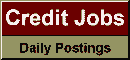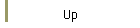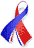DefaultRisk.com the web's biggest credit risk modeling resource.Export citation to:- HTML- Text (plain)- BibTeX- RIS- ReDIF

Dependency without Copulas or Ellipticity

by William T. Shaw of King's College London

September 2007

Abstract: The generation of multivariate probability distributions follows several approaches. Within financial applications the emphasis has mostly been on two methodologies. The first is the elliptical methodology, where the leap from univariate to multivariate has taken place by constructing density functions that are functions of quadratic forms of the marginals. The second is the copula philosophy, where the dependency structure is treated entirely separately from marginals. In financial applications one often needs to work with combinations of marginals of various distributional types, and the copula philosophy is very attractive as it copes well with heterogeneous marginals. However, with some notable exceptions, the copula approach does not normally correspond to any natural or canonical multivariate structure arising from some underlying dynamic.

This paper presents an approach to multivariate distribution theory that allows for heterogeneous marginals but without the limitations of the elliptical ansatz or the arbitrariness of the copula approach. The approach is to consider multivariate distributions as arising naturally from coupled stochastic differential equations (SDEs), and in this paper we consider a first step based on the equilibrium situation. The scope of this paper is where the marginals are one of the classic Pearson family of distributions, allowing for great diversity amongst the marginals. For simplicity we present details for the bivariate case. Using a recently developed quantile form of the Fokker-Planck equation it is first shown how the members of the Pearson family are associated with various types of one-dimensional SDE in a transparent manner. Then appropriate two-dimensional SDEs and the associated Fokker-Planck equations are considered. In the equilibrium limit these give solutions that are natural bivariate structures. Some examples where one marginal is Gaussian or Student are explored. Some possible generalizations are outlined.

Keywords: Dependency, Copula, Elliptical, Stochastic, Fokker-Planck.

Published in: European Journal of Finance, Vol. 15, No. 7-8, (2009), pp. 661-674.

Books Referenced in this paper:  (what is this?)